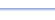Program: TRIRT

TRIRT

• Finds the third side length of a right triangle, given the other two side lengths. (Pythagorean Theorem shortcut!)
• Finds the remaining two sides lengths of a   45-45-90 triangle, given any one side length (45-45-90 Formula shortcut!)
• Finds the remaining two sides lengths of a   30-60-90 triangle, given any one side length (30-60-90 Formula shortcut!)

Useful for:

• SAT
• ACT
• Geometry
• Precalculus
• Trigonometry
• Calculus

See full list of programs

Example 1

Q: Find the value of x:

Solution:

Run TRIRT.  How?

Select choice 1 for
'Pythag'.  This will
do the Pythagorean
Theorem for you.

Choose 2 because
the hypotenuse and
leg are given in the
problem

Enter:
HYP = 10, LEG = 4

The program reports:

Interpretation:
The other leg (x) is 84 or about 9.2.
(To simplify 84 = 221, use the program RADICALN.)

See Example 2